# WO2017034169A1 - Method for transmitting synchronization signal using codebook in wireless communication system - Google Patents

Method for transmitting synchronization signal using codebook in wireless communication system Download PDF

## Info

Publication number
WO2017034169A1
WO2017034169A1 PCT/KR2016/008367 KR2016008367W WO2017034169A1 WO 2017034169 A1 WO2017034169 A1 WO 2017034169A1 KR 2016008367 W KR2016008367 W KR 2016008367W WO 2017034169 A1 WO2017034169 A1 WO 2017034169A1
Authority
WO
WIPO (PCT)
Prior art keywords
plurality
precoders
precoder
synchronization signal
base station
Prior art date
Application number
PCT/KR2016/008367
Other languages
French (fr)
Korean (ko)
Inventor
이길봄
강지원
김기태
박경민
김희진
Original Assignee
엘지전자 주식회사
Priority date (The priority date is an assumption and is not a legal conclusion. Google has not performed a legal analysis and makes no representation as to the accuracy of the date listed.)
Filing date
Publication date
Priority to US201562208798P priority Critical
Priority to US62/208,798 priority
Application filed by 엘지전자 주식회사 filed Critical 엘지전자 주식회사
Publication of WO2017034169A1 publication Critical patent/WO2017034169A1/en

• 238000004891 communication Methods 0 claims description title 33
• 230000000875 corresponding Effects 0 abstract claims description 28
• 238000009740 moulding (composite fabrication) Methods 0 claims description 36
• 230000001360 synchronised Effects 0 claims description 13
• 230000001702 transmitter Effects 0 claims description 11
• 238000000034 methods Methods 0 description 125
• 239000011159 matrix materials Substances 0 description 50
• 101700080873 SYNCI family Proteins 0 description 27
• 230000002829 reduced Effects 0 description 14
• 238000001228 spectrum Methods 0 description 12
• 230000000694 effects Effects 0 description 9
• 239000000969 carrier Substances 0 description 7
• 229920001895 acrylonitrile-acrylic-styrenes Polymers 0 description 6
• 238000004422 calculation algorithm Methods 0 description 6
• 239000002609 media Substances 0 description 6
• 230000015654 memory Effects 0 description 6
• 230000001603 reducing Effects 0 description 5
• 239000006185 dispersions Substances 0 description 4
• 238000003860 storage Methods 0 description 4
• 238000009826 distribution Methods 0 description 3
• 239000000203 mixtures Substances 0 description 3
• 238000006722 reduction reaction Methods 0 description 3
• 101700054812 FUME family Proteins 0 description 2
• 238000007792 addition Methods 0 description 2
• 230000035611 feeding Effects 0 description 2
• 230000014509 gene expression Effects 0 description 2
• 108010003272 hyaluronate lyase Proteins 0 description 2
• 230000001965 increased Effects 0 description 2
• 230000004044 response Effects 0 description 2
• 230000011664 signaling Effects 0 description 2
• 239000000758 substrates Substances 0 description 2
• 101700010516 ALF family Proteins 0 description 1
• 241000760358 Enodes Species 0 description 1
• 239000004676 acrylonitrile butadiene styrene Substances 0 description 1
• 238000004364 calculation methods Methods 0 description 1
• 230000015556 catabolic process Effects 0 description 1
• 230000001413 cellular Effects 0 description 1
• 230000001721 combination Effects 0 description 1
• 230000001276 controlling effects Effects 0 description 1
• 230000004059 degradation Effects 0 description 1
• 238000006731 degradation Methods 0 description 1
• 238000005516 engineering processes Methods 0 description 1
• 238000007667 floating Methods 0 description 1
• 230000001976 improved Effects 0 description 1
• 239000011133 lead Substances 0 description 1
• 239000000463 materials Substances 0 description 1
• 230000000051 modifying Effects 0 description 1
• 230000003287 optical Effects 0 description 1
• 238000005457 optimization Methods 0 description 1
• 230000001151 other effects Effects 0 description 1
• 230000036961 partial Effects 0 description 1
• 239000000047 products Substances 0 description 1
• 230000003595 spectral Effects 0 description 1
• 238000007514 turning Methods 0 description 1

## Images

••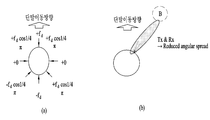••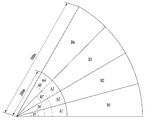•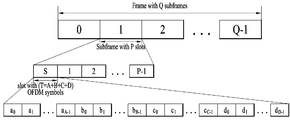•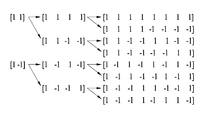•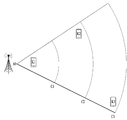•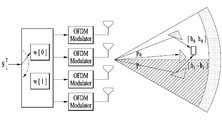•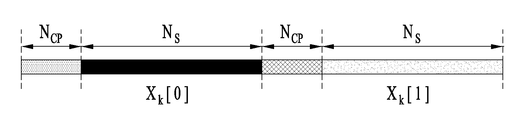•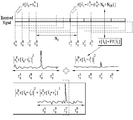••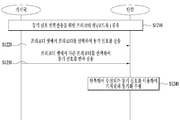•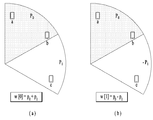••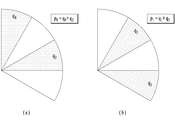•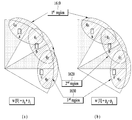•••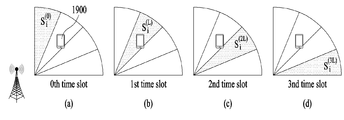•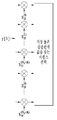•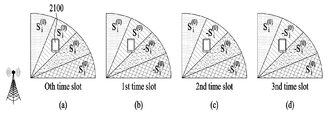•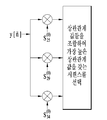••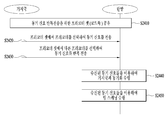•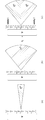•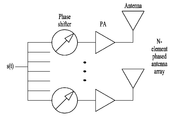•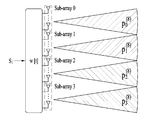•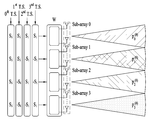••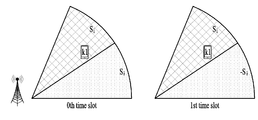•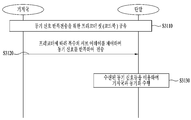•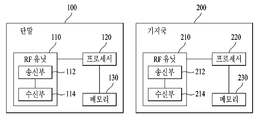## Classifications

• HELECTRICITY
• H04ELECTRIC COMMUNICATION TECHNIQUE
• H04BTRANSMISSION
• H04B7/02Diversity systems; Multi-antenna system, i.e. transmission or reception using multiple antennas
• H04B7/04Diversity systems; Multi-antenna system, i.e. transmission or reception using multiple antennas using two or more spaced independent antennas
• H04B7/06Diversity systems; Multi-antenna system, i.e. transmission or reception using multiple antennas using two or more spaced independent antennas at the transmitting station
• YGENERAL TAGGING OF NEW TECHNOLOGICAL DEVELOPMENTS; GENERAL TAGGING OF CROSS-SECTIONAL TECHNOLOGIES SPANNING OVER SEVERAL SECTIONS OF THE IPC; TECHNICAL SUBJECTS COVERED BY FORMER USPC CROSS-REFERENCE ART COLLECTIONS [XRACs] AND DIGESTS
• Y02TECHNOLOGIES OR APPLICATIONS FOR MITIGATION OR ADAPTATION AGAINST CLIMATE CHANGE
• Y02DCLIMATE CHANGE MITIGATION TECHNOLOGIES IN INFORMATION AND COMMUNICATION TECHNOLOGIES [ICT], I.E. INFORMATION AND COMMUNICATION TECHNOLOGIES AIMING AT THE REDUCTION OF THIR OWN ENERGY USE
• Y02D70/00Techniques for reducing energy consumption in wireless communication networks
• Y02D70/10Techniques for reducing energy consumption in wireless communication networks according to the Radio Access Technology [RAT]
• YGENERAL TAGGING OF NEW TECHNOLOGICAL DEVELOPMENTS; GENERAL TAGGING OF CROSS-SECTIONAL TECHNOLOGIES SPANNING OVER SEVERAL SECTIONS OF THE IPC; TECHNICAL SUBJECTS COVERED BY FORMER USPC CROSS-REFERENCE ART COLLECTIONS [XRACs] AND DIGESTS
• Y02TECHNOLOGIES OR APPLICATIONS FOR MITIGATION OR ADAPTATION AGAINST CLIMATE CHANGE
• Y02DCLIMATE CHANGE MITIGATION TECHNOLOGIES IN INFORMATION AND COMMUNICATION TECHNOLOGIES [ICT], I.E. INFORMATION AND COMMUNICATION TECHNOLOGIES AIMING AT THE REDUCTION OF THIR OWN ENERGY USE
• Y02D70/00Techniques for reducing energy consumption in wireless communication networks
• Y02D70/10Techniques for reducing energy consumption in wireless communication networks according to the Radio Access Technology [RAT]
• Y02D70/12Techniques for reducing energy consumption in wireless communication networks according to the Radio Access Technology [RAT] in 3rd Generation Partnership Project [3GPP] networks
• YGENERAL TAGGING OF NEW TECHNOLOGICAL DEVELOPMENTS; GENERAL TAGGING OF CROSS-SECTIONAL TECHNOLOGIES SPANNING OVER SEVERAL SECTIONS OF THE IPC; TECHNICAL SUBJECTS COVERED BY FORMER USPC CROSS-REFERENCE ART COLLECTIONS [XRACs] AND DIGESTS
• Y02TECHNOLOGIES OR APPLICATIONS FOR MITIGATION OR ADAPTATION AGAINST CLIMATE CHANGE
• Y02DCLIMATE CHANGE MITIGATION TECHNOLOGIES IN INFORMATION AND COMMUNICATION TECHNOLOGIES [ICT], I.E. INFORMATION AND COMMUNICATION TECHNOLOGIES AIMING AT THE REDUCTION OF THIR OWN ENERGY USE
• Y02D70/00Techniques for reducing energy consumption in wireless communication networks
• Y02D70/10Techniques for reducing energy consumption in wireless communication networks according to the Radio Access Technology [RAT]
• Y02D70/12Techniques for reducing energy consumption in wireless communication networks according to the Radio Access Technology [RAT] in 3rd Generation Partnership Project [3GPP] networks
• Y02D70/126Techniques for reducing energy consumption in wireless communication networks according to the Radio Access Technology [RAT] in 3rd Generation Partnership Project [3GPP] networks in 4th generation [4G] networks

## Abstract

Disclosed are a synchronization signal transmission method and a base station, in which beamforming of synchronization signals is performed in the following manner: a codebook is configured by defining a plurality of third precoders by connecting basic precoders which respectively correspond to a plurality of antenna subarrays, the synchronization signals are repeatedly transmitted to a terminal by applying the third precoders selected from the codebook to a synchronization sequence across a plurality of time intervals, and the plurality of antenna subarrays are independently controlled by first precoders respectively corresponding thereto.

## Description

Synchronous Signal Transmission Method Using Codebook in Wireless Communication System

The following description relates to a wireless communication system, and more particularly, to a synchronization signal transmission method and apparatus using a synchronization signal codebook in a WLAN system.

Ultra-high frequency wireless communication systems using millimeter wave (mmWave) are configured such that the center frequency operates at a few GHz to several tens of GHz. Due to the characteristics of the center frequency, path loss may be prominent in the shadow area in the mmWave communication system. Considering that the synchronization signal should be stably transmitted to all terminals located within the coverage of the base station, the mmWave communication system designs and transmits the synchronization signal in consideration of the potential deep-null phenomenon that may occur due to the characteristics of the ultra-high frequency band described above. Should be.

The present invention has been made to solve the above problems, an object of the present invention to improve the synchronization process between the base station and the terminal in the wireless communication system to improve the efficiency of the synchronization process.

Another object of the present invention is to improve the precoder performance applied to a synchronization sequence by proposing a codebook for the transmission of the synchronization signal.

Another object of the present invention is to minimize power loss while maintaining the performance of the synchronization signal transmission process.

Technical objects to be achieved in the present invention are not limited to the above-mentioned matters, and other technical problems not mentioned above are provided to those skilled in the art from the embodiments of the present invention to be described below. May be considered.

According to another aspect of the present invention, there is provided a synchronization signal transmission method including a codebook including second precoders defined as concatenation of a plurality of first precoders corresponding to each of a plurality of antenna subarrays. Acquiring a signal, and repeatedly transmitting a synchronization signal to the terminal by applying different second precoders selected from the codebook over a plurality of time intervals to the synchronization sequence, wherein the plurality of antenna subarrays are assigned to each terminal. Independently controlled by the corresponding first precoder to perform beamforming of the synchronization signal.

One second precoder is defined as a concatenation of a plurality of weighted first precoders, and the weights may be different for each of the plurality of second precoders constituting the codebook.

The weights applied to the plurality of first precoders may have the same magnitude and different phases.

Each of the plurality of first precoders may be defined as a concatenation of third precoders constituting a narrower beam than the first precoder.

The synchronization sequence may remain the same over a plurality of time intervals.

Each of the plurality of antenna subarrays may correspond to an RF chain of a base station.

The base station for solving the technical problem includes a transmitter, a receiver, and a processor operating in connection with the transmitter and the receiver, the processor, a plurality of first free corresponding to each of the plurality of antenna subarrays (antenna subarray) Acquiring a codebook consisting of second precoders defined by concatenation of coders, and applying different second precoders selected from the codebook to the synchronization sequence over a plurality of time intervals, thereby repeating the synchronization signal to the terminal. The plurality of antenna sub-arrays are controlled independently by each other by a first precoder corresponding to each other to perform beamforming of a synchronization signal.

Another synchronization signal transmission method for solving the above technical problem comprises a second precoder defined by concatenation of a plurality of first precoders corresponding to each of a plurality of antenna subarrays. Obtaining a codebook, and applying a second precoder selected from the codebook to a synchronization sequence whose sign is changed every time interval, thereby repeatedly transmitting a synchronization signal to the terminal over a plurality of time intervals. And a plurality of antenna sub-arrays are independently controlled from each other by a first precoder corresponding to each other to perform beamforming of a synchronization signal.

Another base station for solving the technical problem includes a transmitter, a receiver, and a processor operating in connection with the transmitter and the receiver, the processor comprising: a plurality of agents corresponding to each of the plurality of antenna subarrays; By obtaining a codebook consisting of second precoders defined by concatenation of one precoder, and applying any second precoder selected from the codebook to a synchronization sequence whose sign is changed every time interval, Repeatedly transmit the synchronization signal to the terminal over the time interval of, the plurality of antenna sub-arrays are controlled independently of each other by a first precoder corresponding to each, and performs beamforming of the synchronization signal.

According to embodiments of the present invention, the following effects can be expected.

First, the synchronization process between the base station and the terminal in the wireless communication system is improved to enable an efficient synchronization process between the base station and the terminal.

Second, by designing a codebook applied to the synchronization sequence, the precoder performance is improved.

Third, it is to minimize the power loss of the base station in the synchronization process through the Active Antenna System (AAS).

Effects obtained in the embodiments of the present invention are not limited to the above-mentioned effects, and other effects not mentioned above are commonly known in the art to which the present invention pertains from the description of the embodiments of the present invention. Can be clearly derived and understood by those who have In other words, unintended effects of practicing the present invention may also be derived by those skilled in the art from the embodiments of the present invention.

BRIEF DESCRIPTION OF THE DRAWINGS The accompanying drawings are provided to help understand the present disclosure, and provide embodiments of the present disclosure with the detailed description. However, the technical features of the present invention are not limited to the specific drawings, and the features disclosed in the drawings may be combined with each other to constitute a new embodiment. Reference numerals in each drawing refer to structural elements.

1 is a diagram illustrating a Doppler spectrum.

2 is a diagram illustrating narrow beamforming according to the invention.

3 is a diagram illustrating Doppler spectra when narrow beamforming is performed.

4 is a diagram illustrating an example of a synchronization signal service zone of a base station.

5 is an example of a frame structure proposed in a communication environment using mmWave.

6 shows the structure of an Orthogonal Variable Spreading Factor (OVSF) code.

7 is a diagram illustrating an example of an arrangement of terminals.

8 is a diagram illustrating a synchronization signal transmission structure according to one embodiment.

9 illustrates a synchronization signal repeatedly transmitted according to an embodiment.

10 illustrates a process of estimating sequence and timing by a terminal receiving a synchronization signal.

11 illustrates another embodiment of a process in which a terminal synchronizes timing by using a synchronization signal.

12 is a flowchart illustrating a synchronization signal transmission and reception method according to an embodiment.

FIG. 13 is a view illustrating another embodiment related to a synchronization signal transmission / reception method.

14 to 17 are diagrams illustrating a synchronization signal transmission structure according to another embodiment.

18 is a flowchart illustrating a synchronization signal transmission and reception method according to FIGS. 14 to 17.

19 and 20 illustrate a structure of transmitting a synchronization signal and a beam scanning signal for explaining another proposed embodiment.

21 to 23 illustrate a structure of transmitting a synchronization signal and a beam scanning signal according to a proposed embodiment.

24 is a flowchart illustrating a synchronization process and a beam scanning process according to another embodiment of the present disclosure.

25 is a diagram for explaining a beam extension technique.

26 is a diagram illustrating an active antenna system (AAS).

27 to 30 illustrate a synchronization signal and beam scanning signal transmission structure according to another embodiment of the present invention.

31 is a flowchart illustrating a synchronization process and a beam scanning process according to another embodiment of the present disclosure.

32 is a diagram illustrating a configuration of a terminal and a base station according to the proposed embodiment.

The terms used in the present invention have been selected as widely used general terms as possible in consideration of the functions in the present invention, but this may vary according to the intention or precedent of the person skilled in the art, the emergence of new technologies and the like. In addition, in certain cases, there is also a term arbitrarily selected by the applicant, in which case the meaning will be described in detail in the description of the invention. Therefore, the terms used in the present invention should be defined based on the meanings of the terms and the contents throughout the present invention, rather than the names of the simple terms.

The following embodiments combine the components and features of the present invention in a predetermined form. Each component or feature may be considered to be optional unless otherwise stated. Each component or feature may be embodied in a form that is not combined with other components or features. In addition, some of the components and / or features may be combined to form an embodiment of the present invention. The order of the operations described in the embodiments of the present invention may be changed. Some components or features of one embodiment may be included in another embodiment, or may be replaced with corresponding components or features of another embodiment.

In the description of the drawings, procedures or steps which may obscure the gist of the present invention are not described, and procedures or steps that can be understood by those skilled in the art are not described.

Throughout the specification, when a part is said to "comprising" (or including) a component, this means that it may further include other components, except to exclude other components unless specifically stated otherwise. do. In addition, the terms "... unit", "... group", "module", etc. described in the specification mean a unit for processing at least one function or operation, which is hardware or software or a combination of hardware and software. It can be implemented as. Also, "a or an", "one", "the", and the like are used differently in the context of describing the present invention (particularly in the context of the following claims). Unless otherwise indicated or clearly contradicted by context, it may be used in the sense including both the singular and the plural.

In the present specification, embodiments of the present invention have been described based on data transmission / reception relations between a base station and a mobile station. Here, the base station is meant as a terminal node of a network that directly communicates with a mobile station. The specific operation described as performed by the base station in this document may be performed by an upper node of the base station in some cases.

That is, various operations performed for communication with a mobile station in a network consisting of a plurality of network nodes including a base station may be performed by the base station or network nodes other than the base station. In this case, the 'base station' may be replaced by terms such as a fixed station, a Node B, an eNode B (eNB), an advanced base station (ABS), or an access point.

In addition, a 'mobile station (MS)' may be a user equipment (UE), a subscriber station (SS), a mobile subscriber station (MSS), a mobile terminal, an advanced mobile station (AMS), a terminal. (Terminal) or a station (STAtion, STA) and the like can be replaced.

Also, the transmitting end refers to a fixed and / or mobile node that provides a data service or a voice service, and the receiving end refers to a fixed and / or mobile node that receives a data service or a voice service. Therefore, in uplink, a mobile station may be a transmitting end and a base station may be a receiving end. Similarly, in downlink, a mobile station may be a receiving end and a base station may be a transmitting end.

In addition, the description that the device communicates with the 'cell' may mean that the device transmits and receives a signal with the base station of the cell. That is, a substantial target for the device to transmit and receive a signal may be a specific base station, but for convenience of description, it may be described as transmitting and receiving a signal with a cell formed by a specific base station. Similarly, the description of 'macro cell' and / or 'small cell' may not only mean specific coverage, but also 'macro base station supporting macro cell' and / or 'small cell supporting small cell', respectively. It may mean 'base station'.

Embodiments of the present invention may be supported by standard documents disclosed in at least one of the wireless access systems IEEE 802.xx system, 3GPP system, 3GPP LTE system and 3GPP2 system. That is, obvious steps or parts which are not described among the embodiments of the present invention may be described with reference to the above documents.

In addition, all terms disclosed in the present document can be described by the above standard document. In particular, embodiments of the present invention may be supported by one or more of the standard documents P802.16e-2004, P802.16e-2005, P802.16.1, P802.16p, and P802.16.1b standard documents of the IEEE 802.16 system. have.

Hereinafter, exemplary embodiments of the present invention will be described in detail with reference to the accompanying drawings. The detailed description, which will be given below with reference to the accompanying drawings, is intended to explain exemplary embodiments of the present invention and is not intended to represent the only embodiments in which the present invention may be practiced.

In addition, specific terms used in the embodiments of the present invention are provided to help the understanding of the present invention, and the use of the specific terms may be changed to other forms without departing from the technical spirit of the present invention.

1. Communication system using ultra high frequency band

In LTE (Long Term Evolution) / LTE-A (LTE Advanced) system, the error value of the oscillator of the terminal and the base station is defined as a requirement, and is described as follows.

UE side frequency error (in TS 36.101)

The UE modulated carrier frequency shall be accurate to within ± 0.1 PPM observed over a period of one time slot (0.5 ms) compared to the carrier frequency received from the E-UTRA Node B

eNB side frequency error (in TS 36.104)

Frequency error is the measure of the difference between the actual BS transmit frequency and the assigned frequency.

Meanwhile, the oscillator accuracy according to the type of base station is shown in Table 1 below.

Table 1 BS class Accuracy Wide Area BS ± 0.05 ppm Local Area BS ± 0.1 ppm Home BS ± 0.25 ppm

Therefore, the maximum difference of the oscillator between the base station and the terminal is ± 0.1ppm, and when an error occurs in one direction, a maximum offset value of 0.2 ppm may occur. This offset value is multiplied by the center frequency and converted into Hz units for each center frequency.

On the other hand, in the OFDM system, the CFO value is shown differently by subcarrier spacing, and in general, even if the CFO value is large, the effect of the OFDM system with a sufficiently large subcarrier spacing is relatively small. Therefore, the actual CFO value (absolute value) needs to be expressed as a relative value affecting the OFDM system, which is called a normalized CFO. The normalized CFO is expressed by dividing the CFO value by the subcarrier spacing. Table 2 below shows the CFO and normalized CFO for each center frequency and oscillator error value.

TABLE 2 Center frequency (subcarrier spacing) Oscillator offset ± 0.05ppm ± 0.1ppm ± 10 ppm ± 20ppm 2 GHz (15 kHz) ± 100 Hz (± 0.0067) ± 200 Hz (± 0.0133) ± 20 kHz (± 1.3) ± 40 kHz (± 2.7) 30 GHz (104.25 kHz) ± 1.5 kHz (± 0.014) ± 3 kHz (± 0.029) ± 300 kHz (± 2.9) ± 600 kHz (± 5.8) 60 GHz (104.25 kHz) ± 3 kHz (± 0.029) ± 6 kHz (± 0.058) ± 600 kHz (± 5.8) ± 1.2 MHz (± 11.5)

In Table 2, a subcarrier spacing (15 kHz) is assumed for a center frequency of 2 GHz (for example, LTE Rel-8 / 9/10), and a subcarrier spacing of 104.25 kHz for a center frequency of 30 GHz or 60 GHz. This prevents performance degradation considering the Doppler effect for each center frequency. Table 2 above is a simple example and it is apparent that other subcarrier spacings may be used for the center frequency.

On the other hand, in a situation where the terminal moves at a high speed or in a high frequency band, a Doppler spread phenomenon greatly occurs. Doppler dispersion causes dispersion in the frequency domain, resulting in distortion of the received signal at the receiver's point of view. Doppler dispersion

It can be expressed as. In this case, v is the moving speed of the terminal, and λ means the wavelength of the center frequency of the transmitted radio waves. θ means the angle between the received radio wave and the moving direction of the terminal. The following description is based on the assumption that θ is zero.

In this case, the coherence time is in inverse proportion to the Doppler variance. If the coherence time is defined as a time interval in which the correlation value of the channel response in the time domain is 50% or more,

It is expressed as In a wireless communication system, Equation 1 below is mainly used which represents a geometric mean between the equation for Doppler variance and the equation for coherence time.

[Equation 1]

1 is a diagram illustrating a Doppler spectrum.

The Doppler spectrum, or Doppler power spectrum density, which represents a change in Doppler value according to the frequency change, may have various shapes according to a communication environment. In general, in an environment where scattering occurs a lot, such as downtown, if the received signal is received at the same power in all directions, the Doppler spectrum appears in the U-shape as shown in FIG. 1 shows the center frequency

And the maximum Doppler variance U-shaped Doppler spectra are shown.

FIG. 2 is a diagram showing narrow beamforming according to the present invention, and FIG. 3 is a diagram showing Doppler spectrum when narrow beamforming is performed.

Since the ultrahigh frequency wireless communication system is located in a band having a very high center frequency, an antenna array including a plurality of antennas may be installed in a small space with a small antenna. This feature enables pin-point beamforming, pencil beamforming, narrow beamforming, or thin beamforming using tens to hundreds of antennas. This narrow beamforming means that the received signal is received only at a certain angle, not in the same direction.

FIG. 2A illustrates a case where the Doppler spectrum is U-shaped according to a signal received in an equal direction, and FIG. 2B illustrates a case where narrow beamforming using a plurality of antennas is performed.

As such, when the narrow beamforming is performed, the Doppler spectrum also appears narrower than the U-shape due to the reduced angular spread. As shown in FIG. 3, it can be seen that Doppler variance appears only in a certain band when the narrow beamforming is performed.

In the wireless communication system using the ultra-high frequency band described above, the center frequency operates in the band of several GHz to several tens of GHz. This characteristic of the center frequency makes the influence of the CFO due to the Doppler effect or the oscillator difference between the transmitter / receiver caused by the movement of the terminal more serious.

4 is a diagram illustrating an example of a synchronization signal service zone of a base station.

The terminal performs synchronization with the base station by using a downlink (DL) synchronization signal transmitted by the base station. In this synchronization process, timing and frequency are synchronized between the base station and the terminal. In the synchronization process, the base station transmits the synchronization signal by configuring the beam width as wide as possible so that terminals in a specific cell can receive and use the synchronization signal.

On the other hand, in the mmWave communication system using a high frequency band, path loss is greater than that of a low frequency band in synchronizing signal transmission. That is, in the case of a system using a high frequency band, a cell radius that can be supported compared to a conventional cellular system (for example, LTE / LTE-A) using a relatively low frequency band (for example, 6 GHz or less). This is greatly toned.

As one method for solving such a reduction in cell radius, a synchronization signal transmission method using beamforming may be used. When beamforming is used, the cell radius is increased, but the beam width is reduced. Equation 2 below shows the change in the received signal SINR according to the beam width.

[Equation 2]

Equation 2 is the beam width according to the beamforming

In addition to the beamforming method, another method for solving the reduction of the cell radius may be considered to repeatedly transmit the same sync signal. This method requires additional resource allocation on the time axis, but has the advantage of increasing the cell radius without reducing the beam width.

Meanwhile, the base station allocates resources to each terminal by scheduling frequency resources and time resources located in a specific area. Hereinafter, this specific zone is defined as a sector. In the sectors shown in FIG. 4, A1, A2, A3, and A4 represent sectors having a radius of 0 to 200 m and widths of 0 to 15 ', 15 to 30', 30 to 45 ', and 45 to 60', respectively. B1, B2, B3, and B4 represent sectors having a radius of 200 to 500 m and widths of 0 to 15 ', 15 to 30', 30 to 45 ', and 45 to 60', respectively. Based on the contents shown in FIG. 4, sector 1 is defined as {A1, A2, A3, A4}, and sector 2 is defined as {A1, A2, A3, A4, B1, B2, B3, B4}. In addition, if the synchronization signal service area of the current base station is sector 1, it is assumed that an additional power of 6 dB or more is required for transmission of the synchronization signal in order for the base station to service the synchronization signal in sector 2.

First, the base station can obtain an additional gain of 6 dB using the beamforming technique to serve sector 2. Through this beamforming process, the service radius can be increased from A1 to B1. However, since beam width is reduced through beamforming, A2, A3, and A4 cannot be serviced at the same time. Therefore, when beamforming is performed, a synchronization signal should be separately transmitted to the A2 to B2, A3 to B3, and A4 to B4 sectors. In other words, the base station must transmit a synchronization signal four times beamforming to serve sector 2.

On the other hand, considering the repetitive transmission of the synchronization signal described above, the base station can transmit the synchronization signal to all sectors 2, but must transmit the synchronization signal four times on the time axis. As a result, the resources required to service sector 2 are the same for both beamforming and iterative transmission.

However, in the beamforming method, since the beam width is narrow, it is difficult for a terminal moving at a high speed or a terminal at the boundary of a sector to stably receive a synchronization signal. Instead, if the ID of the beam in which the terminal is located can be distinguished, there is an advantage that the terminal can determine its own position through a synchronization signal. On the other hand, in the repetitive transmission scheme, since the beam width is wide, it is very unlikely that the terminal misses the synchronization signal. Instead, the terminal cannot determine its location.

5 is an example of a frame structure proposed in a communication environment using mmWave.

First, one frame consists of Q subframes and one subframe consists of P slots. One slot consists of T OFDM symbols. At this time, unlike the other subframes, the first subframe in the frame uses the 0 th slot (slot indicated by 'S') for synchronization purposes. The 0 th slot is composed of A OFDM symbols for timing and frequency synchronization, B OFDM symbols for beam scanning, and C OFDM symbols for informing the UE of system information. The remaining D OFDM symbols are used for data transmission to each terminal.

Meanwhile, such a frame structure is merely a mere example, and Q, P, T, S, A, B, C, and D may each be arbitrary values and may be values set by a user or automatically set on a system.

Hereinafter, a timing synchronization algorithm between a base station and a terminal will be described. Consider a case in which the base station repeatedly transmits the same synchronization signal A times in FIG. 5. The terminal performs timing synchronization using an algorithm of Equation 3 based on the synchronization signal transmitted from the base station.

[Equation 3]

In equation (3)

, N g , i represent the length of an OFDM symbol, the length of a cyclic prefix (CP), and the index of an OFDM symbol, respectively. Denotes a vector of the received signal at the receiver. At this time, Cold signal vector of From the first Vector defined by the first element.

The algorithm of Equation 3 operates under the condition that two adjacent OFDM received signals in time are the same. Such an algorithm can use a sliding window method, which can be implemented with low complexity, and has a strong characteristic of frequency offset.

Meanwhile, Equation 4 below represents an algorithm for performing timing synchronization by using a correlation between a received signal and a signal transmitted by a base station.

[Equation 4]

In Equation 4, s denotes a signal transmitted by the base station and is a signal vector previously promised between the terminal and the base station. Equation 4 may produce better performance than Equation 3, but may not be implemented as a sliding window method, and thus requires high complexity. It also has a feature that is vulnerable to frequency offset.

Following the description of the timing synchronization method, the beam scanning process will be described. Beam scanning refers to the operation of the transmitter and / or receiver to find the direction of the beam that maximizes the receiver's received SINR. For example, the base station determines the direction of the beam through beam scanning before transmitting data to the terminal.

Referring to FIG. 4 as an example, FIG. 4 illustrates a sector served by one base station divided into eight regions. At this time, the base station transmits beams in the areas (A1 + B1), (A2 + B2), (A3 + B3), and (A4 + B4), respectively, and the terminal can distinguish beams transmitted by the base station. In this condition, the beam scanning process can be embodied in four processes. First, i) the base station transmits a beam in four areas in sequence. ii) The terminal determines the beam that is determined to be the most suitable among the beams in view of the received SINR. iii) The terminal feeds back information on the selected beam to the base station. iv) The base station transmits data using the beam having the feedback direction. Through the above beam scanning process, the UE can receive downlink data through the beam with optimized reception SINR.

Hereinafter, the Zadoff-Chu sequence will be described. The Zadoff-Chu sequence is called a chu sequence or ZC sequence and is defined by Equation 5 below.

[Equation 5]

In Equation 5, N is the length of the sequence, r is the root value,

Represents the n th element of the ZC sequence. A characteristic of the ZC sequence is that all elements have the same size (constant amplitude). In addition, the DFT results of the ZC sequence also appear the same for all elements.

Next, the ZC sequence and the cyclic shifted version of the ZC sequence have a correlation as shown in Equation (6).

[Equation 6]

In equation (6)

Is Is a sequence of circular shifted by i, and represents 0 except that the autocorrelation of the ZC sequence is i = j. In addition, the ZC sequence also has a zero auto-correlation property, it is also expressed as having a constant Amplitude Zero Auto Correlation (CAZAC).

As a final feature of the ZC sequence, there is a correlation between the length N of the sequence and the ZC sequences having root values that are mutually smaller than Equation 7 below.

[Equation 7]

In equation (7)

Is mutually different from N. For example, if N = 111 Always satisfies equation (7). Unlike the autocorrelation of Equation 6, the cross-correlation of the ZC sequence is not completely zero.

Following the ZC sequence, the Hadamard matrix is described. Hadamard matrix is defined as Equation 8 below.

[Equation 8]

In equation (8)

Denotes the size of the matrix. Hadamard matrices are always independent of size n Is a unitary matrix that satisfies. Also, in the Hadamard matrix, all columns and all rows are orthogonal to each other. For example, when n = 4, the Hadamard matrix is defined as in Equation 9.

[Equation 9]

It can be seen from Equation 9 that the columns are orthogonal to each other.

6 shows the structure of an Orthogonal Variable Spreading Factor (OVSF) code. The OVSF code is generated based on the Hadamard matrix and has a specific rule.

First, when branching to the right side of the OVSF code (lower branch), the first code repeats the upper code on the left side twice (mother code), and the second code repeats the high code code once and inverts it once. Is generated. 6 shows a tree structure of the OVSF code.

All of these OVSF codes are orthogonal except for the relationship between adjacent higher and lower codes on the code tree. For example, in FIG. 6, the code [1 -1 1 -1] is orthogonal to [1 1], [1 1 1 1], and [1 1 -1 -1]. In addition, the OVSF code has the same length as the code length. That is, in FIG. 6, it can be seen that the length of a specific code is equal to the total number of branches to which the corresponding code belongs.

7 is a diagram illustrating an example of an arrangement of terminals. In FIG. 7, a random access channel (RACH) will be described.

In the LTE system, when the RACH signals transmitted by the terminals arrive at the base station, the RACH signal power of the terminals received by the base station should be the same. To this end, the base station defines a parameter called 'preambleInitialReceivedTargetPower', and broadcasts the parameter to all terminals in the cell through SIB (System Information Block) 2. The UE calculates a path loss using a reference signal, and determines the transmission power of the RACH signal by using the calculated path loss and the 'preambleInitialReceivedTargetPower' parameter as shown in Equation 10 below.

[Equation 10]

In Equation 10, P_PRACH_Initial, P_CMAX, and PL represent the transmission power of the RACH signal, the maximum transmission power of the terminal, and the path loss, respectively.

Referring to Equation 10 as an example, it is assumed that the maximum transmit power of the terminal is 23 dBm and the RACH reception power of the base station is -104 dBm. In addition, it is assumed that the terminal is arranged as shown in FIG.

First, the terminal calculates a path loss using the received synchronization signal and the beam scanning signal, and determines the transmission power based on this. Table 3 below shows the path loss of the terminal and its transmission power.

TABLE 3 Terminal preambleInitialReceivedTargetPower Path loss Transmission power required Transmission power Extra power needed K1 -104dBm 60 dB -44 dBm -44 dBm 0 dBm K2 -104dBm 110 dB 6 dBm 6 dBm 0 dBm K3 -104dBm 130 dB 26 dBm 23 dBm 3 dBm

In Table 3, although the path loss is very small in the case of the K1 terminal, the RACH signal must be transmitted with a very small power (-44 dBm) to match the RACH reception power. Meanwhile, in the case of the K2 terminal, the path loss is large, but the required transmission power is 6 dBm. However, in the case of the K3 terminal, the path loss is so large that the required transmission power exceeds the P_CMAX = 23 dBm of the terminal. In this case, the terminal should transmit at 23 dBm, which is the maximum transmission power, and the RACH access success rate of the terminal is degraded by 3 dB.

2. Proposed synchronization signal transmission and reception method 1

8 is a diagram illustrating a synchronization signal transmission structure according to an exemplary embodiment. According to the proposed embodiment, the base station defines a new precoder consisting of a weighted sum of two or more basic precoders applied to a repeatedly transmitted synchronization signal. The base station also defines a plurality of new precoders generated by changing the weighted sum as a codebook.

In FIG. 8, a case where the number of repetitive transmissions of the synchronization signal is 2 is illustrated, and a repetitive transmission structure of the synchronization signal for obtaining diversity is illustrated.

First, the number of antennas, the length of an OFDM symbol, and the length of a CP of a base station transmitting a synchronization signal

It is called. In addition, each of the basic precoder We define a new precoder It is defined as In the basic precoder and the new precoder, '0' and '1' represent an order in which a synchronization signal is transmitted, that is, an OFDM symbol. The basic precoder and the new precoder are vector matrices, the size of which is equal to the number of antenna ports of the base station. That is, in FIG. And The size of is 4x1 vector.

Meanwhile, in the embodiment of FIG. 8, the codebook defined by the base station is

Expressed as a codebook May be understood as Equation 11 below.

[Equation 11]

Precoder in Equation 11

Is the default precoder Precoder Is the default precoder It consists of a difference. In other words, the new precoder Is defined as the weighted sum of the two basic precoders and the weights of the weighted sums are different. Taking the cell structure shown on the right side of FIG. 8 as an example, the precoder corresponding to the upper subsector is , The precoder for the lower subsector Are each defined as At this time, the base station is a new precoder defined by the weighted sum [+1 +1] in the first OFDM symbol The new signal is defined as another weighted sum [+1 -1] in the second OFDM symbol. Apply the signal to transmit the synchronization signal. Accordingly, even if the terminal is located at the subsector boundary, it is possible to obtain diversity for the two precoders, thereby accurately distinguishing the synchronization signal.

In the above description, it is assumed that the number of repetitions is two. On the other hand, the number of times of repeated transmission of the synchronization signal of the base station

In this case, the precoder codebook of the synchronization signal is generalized and defined as in Equation 12 below.

[Equation 12]

Vector from Equation 12

Denotes a precoder applied to the synchronization signal repeatedly transmitted t-th. The sync signal codebook A matrix, defined as, that represents a channel Is the size Is an arbitrary matrix

That is, in the process of repeatedly transmitting a synchronization signal, the base station selects any one of the codebooks consisting of a weighted sum of basic precoders and a plurality of different new precoders and applies them to the synchronization signal at each repetition. do. The number of new precoders included in the codebook may be equal to the number of repetitive transmissions of synchronization signals of the base station.

9 illustrates a synchronization signal repeatedly transmitted according to the proposed embodiment.

Meanwhile, according to another exemplary embodiment, in the process of repeatedly transmitting the synchronization signal by the base station, the base station selects a precoder that has not been selected in the codebook and transmits the synchronization signal every repetition. That is, in order to maximize the transmission diversity of the synchronization signal, the base station performs a codebook every repetition of the synchronization signal.

Contained in Select a precoder that has not been selected among the two precoders.

For example, in the case of Equation 11 having a repetition number of 2, the codebook

When the base station transmits a synchronization signal in the first OFDM symbol Is selected, and the precoder is used to transmit a synchronization signal in the subsequent second OFDM symbol. Select. This process is shown in the structure in which the switch of the RF module is changed on the left side of FIG. At this time, Sync signals transmitted through the k th antenna among the 4 transmit antennas are the i th sequence And precoder By using Equation 13 is generated as follows.

[Equation 13]

In equation (13)

Represents an Inverse Discrete Fourier Transform (IDFT) matrix, Is Represents the k th element of the precoder. FIG. 9 illustrates a process in which a synchronization signal in the k-th antenna described in Equation 13 is repeatedly transmitted twice.

10 illustrates a process of estimating sequence and timing by a terminal receiving a synchronization signal. 10 illustrates an operation of a terminal side when a base station repeatedly transmits a synchronization signal according to the embodiment described above.

First, an algorithm for synchronizing timing and estimating a sequence from a received synchronization signal may be expressed by Equation 14 below.

[Equation 14]

In equation (14)

Are trial numbers used in estimating timing and sequence, respectively. Denotes a trial number when the value of Equation 14 is maximized, and denotes an index of a timing and a sequence estimated from a synchronization signal received by the terminal.

On the other hand, Equation 14 may be represented as shown in FIG. Four different timings in Figure 10

Is shown, and the magnitude of the correlation between the received signal and the sequence at each time point is shown. Also, in FIG. 10 Is Represents the received signal vector received at the time point, and the length to be. Is Means the signal after the DFT processing.

Referring to Figure 10, the terminal each timing

And sequence index Equation 14 is calculated by applying as a trial number. In the example of FIG. 10, the terminal calculates the result of equation (14). Since the correlation value calculated by is the largest, the terminal Is determined as the timing of the synchronization signal, and it is determined that the i th sequence is transmitted.

On the other hand, if the channel does not change while the synchronization signal is repeatedly received two times and there is no noise, the magnitude of the peak value calculated by the UE by Equation 14 is expressed by Equation 15 below.

[Equation 15]

In equation (15)

Is, Denotes a channel between the transmitter and the receiver. The received SNR of the UE from Equation 15 is calculated as in Equation 16 below.

[Equation 16]

In equation (16)

Denotes a reception SNR when simply transmitting a synchronization signal repeatedly, and SNR proposed represents a reception SNR when the synchronization signal is transmitted by configuring a precoder to obtain diversity according to the proposed embodiment. The former doubles the receive power of the sync signal, while the latter receives two channels. And It is expressed as if And If is independent of each other, the diversity gain of the SNR proposed becomes 2. In contrast, Receive power is one channel Since it is expressed only as, the diversity gain is one.

On the other hand, since the synchronization signal must be detectable by all terminals in the cell, the most important factor in the synchronization signal is the stability of the communication link. Considering that the higher the diversity gain, the higher the stability of the communication link, the proposed embodiment can obtain an improved effect compared to the conventional synchronization signal transmission method.

11 illustrates another embodiment of a process in which a terminal synchronizes timing by using a synchronization signal.

As described above with reference to FIG. 10 and Equation 15,

The peak value at that point Is calculated. Meanwhile, The peak value when is Is calculated. Term from two peak values It can be seen that this is common.

Therefore, according to the proposed embodiment, the terminal

Obtained in the process of calculating the peak value at the time point Store the values on the stack, The stored value can be used to calculate the peak value at the time point. As a result, the UE can reduce the computational complexity by avoiding duplicate calculations by utilizing the memory.

The foregoing is shown in FIG. In Figure 11

Is The terminal is currently obtained in the process of calculating the peak value A process of storing a value on the stack (S1120) is defined as a 'push' operation, and a process of loading and using a pre-stored value from the stack (S1110) is defined as a 'pop up' operation. In FIG. 11, as the 'push' operation and the 'pop up' operation are performed in pairs, the total size of the stack is always Is maintained.

On the other hand, the embodiment using the stack as described above, the number of random repetition

The stack is defined as in Equation 17 below and the size is Becomes That is, the UE stores the calculated values on the stack for the time interval of the length of the OFDM symbol plus the length of the CP, and recalls the stored values when the time interval corresponding to the length of the OFDM symbol and the length of the CP is over. And new calculations.

[Equation 17]

12 is a flowchart illustrating a proposed synchronization signal transmission and reception method. In FIG. 12, the previously proposed and described embodiments are illustrated and described according to a time series flow. Therefore, the above descriptions may be applied to the same or similarly, although not explicitly described in FIG. 12.

First, a precoder set (ie, codebook) for repeatedly transmitting a synchronization signal is shared between a base station and a terminal (S1210). This codebook consists of a plurality of new precoders composed of weighted sums of basic precoders, and the weighted sum is applied differently to each new precoder. Meanwhile, the codebook may be generated by the base station and transmitted to the terminal, or the terminal may directly generate the codebook.

Subsequently, the base station selects any one of the precoders constituting the precoder set (codebook) and transmits a synchronization signal (S1220). As the precoder applied to the synchronization signal, any one of the precoders included in the precoder set may be arbitrarily selected. Subsequently, the base station selects another precoder from the precoder set (codebook) in the next OFDM symbol and repeatedly transmits a synchronization signal (S1230). In S1230, any one of the precoders other than the precoder selected in S1220 is selected among the precoder sets. In FIG. 12, it is assumed that the number of repetitive transmissions of the synchronization signal is 2, but when the number of repetitions is higher, the process of S1230 may be repeatedly performed.

The terminal performs synchronization with the base station by using the synchronization signal repeatedly received (S1240). Such a process may be understood as a process of estimating an optimal value by calculating a correlation between a timing and a sequence of a received synchronization signal, and storing and loading intermediate values on a stack in the process of calculating the correlation. Embodiments may be applied.

According to the embodiments described above, as the transmission diversity is obtained in the process of repeatedly transmitting the synchronization signal, the stability of the communication link is secured. Therefore, in a communication environment in which path loss is large, such as an mmWave communication system, a synchronization signal may be stably transmitted to a terminal.

3. Proposed synchronization signal transmission and reception method 2

13 illustrates a synchronization signal transmission structure according to FIGS. 8 to 12. The reception SNR described in Equation 16 may be expressed as Equation 18 below in consideration of path attenuation.

Equation 18

In equation (18)

Is Path attenuation when the beam reaches the terminal.

Meanwhile, in the case of UE b in FIG. 13, since it is located at two subsector boundaries,

By receiving all the beams Becomes In this environment, the received SNR for the b terminal may be approximated as shown in Equation 19 below.

[Equation 19]

Equation 19 means that the diversity gain for the terminal b can be obtained as two.

On the other hand, a terminal is

Because it is located far from Becomes In consideration of such an environment, the reception SNR of the UE a is approximated by Equation 20 below.

[Equation 20]

Equation 20 means that the diversity gain obtained by terminal a is 1 rather than 2. c terminal is reversed

Located far from, similar to terminal a, only one diversity gain can be obtained. In other words, according to the embodiments described with reference to FIGS. 8 to 12, sufficient diversity gain can be obtained in the case of terminal b, but not in the case of terminals a and c.

The terminal must receive the synchronization signal with a certain quality or higher regardless of the position in the cell. Therefore, hereinafter, an embodiment for improving the point that the UEs obtain different diversity gains according to the position in the cell as described above is proposed.

Among the contents described above with reference to FIGS. 8 to 12, the 'basic precoder

'Suggest ways of designing. Hereinafter, the 'secondary precoder' is the basic precoder described above. Means. According to an embodiment of the present disclosure, the secondary precoder may include two or more 'primary precoders ( Is composed of weighted summation 8 and 12, the plurality of primary precoders ( By the weighted sum of ) Is defined and a new precoder (hereinafter referred to as tertiary precoder) by weighted sum of a plurality of secondary precoders Is defined.

the j th primary precoder and the i th secondary precoder

, When expressed as, the relationship between two precoders is expressed according to Equation 21 below.

[Equation 21]

In Equation 21

Denotes the weights of the primary precoders constituting the i-th secondary precoder as a complex number, that is, how the primary precoders are weighted. Denotes the number of primary precoders constituting the i th secondary precoder, Denotes the index of the secondary precoders constituting the i-th secondary precoder.

Equation 22 below shows an example in which the secondary precoders are composed of a weighted sum of two primary precoders.

[Equation 22]

In Equation 22

ego, ego, to be. Since is a complex number, the secondary precoder may be implemented in a form in which a specific primary precoder is multiplied by a j or -j value that changes a phase.

14 illustrates an example in which primary precoders constituting the secondary precoder are designed.

According to an embodiment, the primary precoders may be designed to equally divide the area where the synchronization signal is transmitted. In the embodiment shown in FIG. 14, the primary precoders are designed such that each beam is formed by 30 'to equally divide 120' which is a synchronization signal transmission region.

In this case, the primary precoders may be designed such that a minimum chordal distance between each other is maximized. The minimum codal distance means the spacing between beams by the precoder, and the maximum codal distance means the maximum spacing between the beams formed by the precoders, that is, the correlation between the beams is minimized. As an example, the primary precoder may be designed to maximize the minimum codal distance using the DFT codebook.

15 to 17 are diagrams illustrating a synchronization signal transmission structure according to another embodiment. Hereinafter, embodiments of designing a second precoder using a first precoder in addition to the above descriptions will be described.

According to an embodiment, the secondary precoder is composed of a weighted sum of the primary precoders and may be designed in a comb structure. The comb structure means that the regions in the subsectors of the primary precoders constituting the secondary precoder are not neighboring as shown in FIG. 14, and the minimum distances of the regions in the subsectors of the primary precoders are the same.

For example, the secondary precoder in Fig. 15 (a)

Primary precoder to construct Wow The regions of do not neighbor each other, and the secondary precoder in FIG. Primary precoder to construct Wow The areas of do not neighbor each other. Furthermore, the second precoder Primary Precoders Composing Wow The minimum distance of the region in the subsector of 30 'is the second precoder Primary Precoders Composing Wow The minimum distance of the region in the subsector of is also equal to 30 '. 2nd precoder Beam area of the secondary precoder It may be understood that the beam area of P is shifted by 30 '.

Meanwhile, when the secondary precoder is configured using the primary precoder according to the above-described embodiment, the third precoders of Equation 11 may be expressed as Equation 23 below.

[Equation 23]

In equation (23)

, Looking at the sign of, it can be seen that the phase of the synchronization signal is inverted with respect to some region in the subsector in the second time interval.

16 illustrates a case where a secondary precoder is designed according to an exemplary embodiment. As described above in Equation 23, during two time periods,

, The phase of is reversed. On the other hand, when the phase change of the beam for each time interval is described for each of the regions 1610, 1620, and 1630 shown, the phase of the beam is changed over the first time interval and the second time interval for the region 1610. To change. For the region 1620, the beam phase over a total of two time intervals For the area 1630 To change.

Unlike the case of FIG. 13, the terminal a of the embodiment of FIG. 16 is adjacent to the beam over two time intervals.

Undergoes a phase change. Accordingly, the terminal a may obtain a diversity gain of 2 for the synchronization signal. Similarly, terminal b is a beam Undergoes a phase shift of By undergoing a phase change of, all terminals in the subsector can obtain the same diversity gain.

FIG. 17 illustrates an embodiment of narrower design of an area within a subsector corresponding to primary precoders. In FIG. 14 to FIG. 16, four primary precoders are assumed, while in FIG. 17, a secondary precoder is designed through eight primary precoders. According to the embodiment proposed in FIG. 17, the terminal receiving the synchronization signal may obtain a more uniform diversity gain than in the embodiment of FIGS. 14 to 16.

18 is a flowchart illustrating a synchronization signal transmission and reception method according to another embodiment. In FIG. 18, the previously proposed and described embodiments are illustrated and described according to a time series flow. Therefore, the above descriptions may be applied to the same or similarly, although not explicitly described in FIG. 18.

First, a precoder set (ie, a codebook) for repeatedly transmitting a synchronization signal is shared between a base station and a terminal (S1810). Such a codebook may consist of tertiary precoders composed of weighted sums of secondary precoders, and the weighted sum is applied differently to each secondary precoder. On the other hand, the secondary precoders are composed of weighted sums of other primary precoders, and the secondary precoders do not have neighboring regions in the subsectors of the primary precoders constituting each secondary precoder, and the primary The minimum distances of the regions in the subsectors of the precoders may be designed in the same comb structure. Meanwhile, the codebook may be generated by the base station and transmitted to the terminal, and the terminal may directly generate the codebook as described above with reference to FIG. 12.

Subsequently, the base station selects any one of the third precoders constituting the precoder set (codebook) and transmits a synchronization signal (S1820). As the third precoder applied to the synchronization signal, any one of the third precoders included in the precoder set may be arbitrarily selected. Subsequently, the base station selects another third-order precoder of the precoder set (codebook) in the next OFDM symbol and repeatedly transmits a synchronization signal (S1830). In S1830, any one of the precoder sets, except for the precoder selected in S1820, is selected. In FIG. 18, it is assumed that the number of repetitive transmissions of the synchronization signal is 2, but when the number of repetitions is higher, the process of S1830 may be repeatedly performed.

The terminal performs synchronization with the base station using the repeatedly received synchronization signal (S1840). This process may be understood as a process of estimating an optimal value by calculating a correlation between the timing and the sequence of the received synchronization signal. In addition, in the process of S1840, according to the embodiment described above with reference to FIGS. 10 and 11, an embodiment in which the terminal stores and retrieves intermediate values in a stack may be applied in the process of calculating correlation.

According to the embodiments described above, as the transmission diversity is obtained in the process of repeatedly transmitting the synchronization signal, the stability of the communication link is secured. In addition, the synchronization signal may be transmitted to the terminals in the cell with a constant diversity gain without additional signaling overhead.

4. Proposed synchronization process and beam scanning process

19 and 20 illustrate a structure of transmitting a synchronization signal and a beam scanning signal for explaining another proposed embodiment. 19 and 20 will be described a synchronization process and a beam scanning process according to the above-described synchronization signal transmission and reception method.

19 illustrates a process of a base station switching four beams during four time slots and transmitting a synchronization signal. The terminal performs a synchronization process using the received synchronization signals, and simultaneously performs a beam scanning process. For example, the terminal 1900 performs synchronization through the second beam (FIG. 19 (b)) in which the reception power is sensed the largest, and the base station can determine that the beam selected by the terminal can be distinguished from other beams. Map beams in different sequences. As a result, the terminal 1900 has a sequence of beams selected by itself.

It can be seen that the beam scanning process is performed by feeding back the selected beam to the base station.

In this process, the signal transmitted by the base station is designed based on the Zadoff-Chu (ZC) sequence. The cell ID of the base station may be used as a root value of the ZC sequence, and the beam ID may be used as a cyclic shift value of the ZC sequence. For example, a sequence

The root value in And the cyclic shift value is Defined as At this time, Is a system parameter value and is generally set to be greater than the channel's delay spread maximum. Terminal sequence Using three cells and four beams of each cell can be distinguished, and this process is expressed by Equation 24 below.

[Equation 24]

The terminal simultaneously performs timing synchronization, cell ID estimation, and beam ID estimation through Equation 24, and this process is represented in FIG.

According to FIG. 20, the terminal is configured to include a total of 12 correlators in order to simultaneously distinguish three cells and four beams, and 12 correlators operate together in every time slot. In the conventional LTE, since only the cell needs to be distinguished, the terminal is configured to include a total of three correlators. On the other hand, the mmWave communication system includes a beam scanning process, requiring four times the processing power of LTE. As a result, according to the scheme proposed above, the computational complexity required for the synchronization process of the terminal is increased four times.

Therefore, hereinafter, an embodiment for improving the complexity of the terminal in the synchronization process and the beam scanning process is proposed. 21 to 23 illustrate a structure of transmitting a synchronization signal and a beam scanning signal according to a proposed embodiment. According to the proposed embodiment, the beam ID is defined as weights applied to the weighted sum of the aforementioned basic precoder (or the secondary precoder).

A case where the number of repetition of the synchronization signal of the base station is 2 is described as an example. Equation 25 below shows a synchronization signal codebook when the number of repetitions is two.

[Equation 25]

Equation 25 uses the codebook described in Equation 11 as a weighting matrix (

). First column in equation (25) Each element of Is the primary precoder (secondary precoder) Represents the weight over time. Similarly, the second column Each element of the second precoder Represents the weight over time. The base station and the terminal , Each Beam ID and Is determined by the beam ID. In other words, each column constituting a codebook (that is, a weight matrix) applied to the transmission of the synchronization signal is used as a beam ID for distinguishing each beam in the beam scanning process. That is, since the base station can transmit the beam ID to the terminal through the weight matrix, the base station can deliver the beam ID for the beam scanning process to the terminal even without additional signaling.

Similarly, the case where the number of repetition of the synchronization signal is 4 will be described using Equation 26 as an example.

[Equation 26]

Equation (26) is a representation of the codebook when the number of repetitions of the synchronization signal is 4 by a weight matrix. Similar to the equation (25), the first, second, third and fourth columns of the weight matrix

Are defined as beam IDs.

Hereinafter, a process of performing beam scanning by a terminal according to an exemplary embodiment will be described in detail. In FIG. 21, a case where the number of repetition of the synchronization signal is 4 and Equation 26 is applied will be described as an example.

According to the exemplary embodiment described with reference to FIGS. 8 to 12, each beam is transmitted as shown in FIG. 21 during four timeslots. In FIG. 21, unlike FIG. 19, four beams are simultaneously transmitted in each timeslot, and the sign of the transmitted sequence varies according to the timeslot. In addition, in FIG. 21, beams transmitted to respective regions within a sector are directed from the top to the bottom.

Corresponds to each. That is, the beam transmitted to the uppermost region And the beam transmitted in the lowest region Corresponds to.

As shown in FIG. 21, when the synchronization signal is transmitted, the terminal performs synchronization according to Equation 27 below to estimate timing synchronization and a sequence (cell ID) of the synchronization signal. Unlike Equation 24, in Equation 27, the beam scanning process for estimating the beam ID is not performed simultaneously with the synchronization process.

[Equation 27]

When the terminal performs only synchronization according to Equation 27, the number of correlators of the terminal is reduced to 4 as compared to 12 of FIG. 20 as shown in FIG. As the number of correlators is reduced, the computational complexity of the terminal is greatly reduced, which may solve the above-described problem.

Meanwhile, the following describes a process of performing beam scanning after the terminal performs synchronization to estimate timing synchronization and sequence.

Each of the timing and sequence indices determined according to Equation 27,

Expressed as Also, The correlation value between the received signal and the sequence obtained in the process of It is defined as At this time, if there is no noise, the correlation value calculated for each timeslot May be expressed as Equation 28 below.

[Equation 28]

The terminal performs a beam scanning process based on Equation 28. First, by multiplying both sides of Equation 28 by the inverse of the weight matrix, Equation 29 may be obtained.

[Equation 29]

In Equation 29, the result calculated through the matrix operation is represented by Equation 30 below.

Equation 30

The terminal compares four power values obtained according to Equation 30, and selects the largest value. For example, the element of the first row

If the value appears to be the largest, the terminal end It can be understood as larger. That is, the terminal in FIG. It can be seen that the beam of the first region generated by is received more strongly than the beams of the other regions. In other words, the terminal may know that it is located in the first area within the sector. That is, the terminal Value is the first column of the weight matrix This is because it knows that the value is calculated using, and as the codebook is shared between the base station and the terminal end This is because the UE knows in advance that it is mapped to. That is, since the UE knows in advance the beam ID mapped to the column selected from the weight matrix, the beam matrix may be performed even if the beam ID is not separately received by calculating the weight matrix.

In another example, an element in Equation 30

If the value of appears to be the largest, the terminal You can see that the value is the largest. That is, the terminal in FIG. It can be seen that the beam of the last region generated by is received more strongly than the beam of the other region. Accordingly, the terminal has a position in its sector It can be seen that the last region corresponding to.

According to the above-described process, even if the base station does not separately generate and allocate the beam ID for the beam scanning process, the terminal may distinguish the beams by using the weight matrix of the synchronization signal repeatedly received (that is, the beam scanning process may be May be performed).

According to the embodiment described above, even if a sequence for the beam ID is not newly defined in the beam scanning process, the terminal may distinguish the beams. Accordingly, the number of sequences to be found by the terminal in timing estimation is reduced by the number of cell IDs, and the synchronization complexity of the terminal is greatly reduced.

According to an embodiment, in the proposed embodiment, the columns of the weight matrix may be configured to be orthogonal to each other. Equations 25 and 26 represent weight matrices when the number of repetitions is 2 or 4, respectively. As such, when the columns of the weight matrix are designed to be orthogonal to each other, a process of applying an inverse of the weight matrix in Equation 28 may be simplified.

The columns of the weighting matrix are configured to be orthogonal to each other, meaning that the vector representing the weighted sum applied to the primary (secondary) precoder in one time slot is orthogonal to the vector representing the weighted sum applied to another time slot. it means. This means that the sync signal codebook

Each vector constituting It may be understood that codebooks are designed to allow each other to work together. Such a vector may be understood as an orthogonal cover code (OCC) in that codes are orthogonal to each other.

In order for each vector to be orthogonal to each other, the codebook may be designed using a Hadamard matrix described in Equation 8, or may be designed using a DFT matrix. The Hadamard matrix can be simply generated for any number of iterations, and the implementation complexity is very low because the values of each element are defined by addition and subtraction only. The DFT matrix can also be simply generated for any number of iterations, where the values of each element

(N is the number of repetitions). For example, the DFT matrix when the number of repetitions is 4 may be generated as in Equation 31 below.

Equation 31

In the case of the DFT matrix, if the number of repetitions is 2, the complexity is the same as that of the Hadamard matrix. If the number of repetitions is 4 or less, the DFT matrix can be generated simply by changing the phase. The rise can be minimized.

In FIG. 23, an embodiment different from FIGS. 21 and 22 will be described. According to the embodiment shown in FIG. 23, the beam ID may be defined by both a weight and a sequence applied to the basic precoder. According to the embodiments described with reference to FIGS. 21 and 22, when the channel changes rapidly while the synchronization signal is repeatedly transmitted, the performance of beam scanning may be degraded. As a method for minimizing such performance degradation, the embodiment illustrated in FIG. 23 proposes a method of generating a beam ID by considering not only a weight matrix but also a sequence.

23 (a) and 23 (b), the base station performs two consecutive timeslots.

Are transmitted simultaneously, and in FIG. 23 (c) and FIG. 23 (d), the base station Send simultaneously.

At this time,

To distinguish them from each other Each of the weights applied to Defined as Likewise, To distinguish them from each other Each of the weights applied to Defined as In this case, the terminal through the process described in Equation 28 to Equation 30 Wow Can be identified separately.

Meanwhile, the base station is transmitted in the first two timeslots

Transmitted in the two timeslots In order to distinguish between them, the first two timeslots contain a sequence ((A) and (b)), and in the following two timeslots, the sequence Are allocated (Figs. 23 (c) and 23 (d)). In this case, since an additional sequence is allocated to distinguish between the upper two regions and the lower two regions in the sector, the number of correlators of the terminal increases by that amount.

According to the embodiment described with reference to FIG. 23, the section in which the channel should not be changed is reduced to two timeslots, thereby making it more robust to sudden changes in the channel.

24 is a flowchart illustrating a synchronization process and a beam scanning process according to another embodiment of the present disclosure. In FIG. 24, the previously proposed and described embodiments are illustrated and described according to a time series flow. Therefore, although not explicitly described with reference to FIG. 24, the contents described above with reference to FIGS. 19 to 23 may be identically or similarly applied.

First, a precoder set (ie, a codebook) for repeatedly transmitting a synchronization signal is shared between a base station and a terminal (S2410). Such a codebook may consist of tertiary precoders composed of weighted sums of secondary precoders, and the weighted sum is applied differently to each secondary precoder. In this case, each of the weighting vectors (ie, OCCs) used for generation of the third order precoders constituting the precoder set may be configured to be orthogonal to each other. Meanwhile, the codebook may be generated by the base station and transmitted to the terminal, and the terminal may directly generate the codebook as described above with reference to FIG. 12. In this process, the weighted sum applied to each tertiary precoder indicates the beam ID of the base station.

Subsequently, the base station selects any one of the third precoders constituting the precoder set (codebook) and transmits a synchronization signal (S2420). As the third precoder applied to the synchronization signal, any one of the third precoders included in the precoder set may be arbitrarily selected. Subsequently, the base station selects another third-order precoder of the precoder set (codebook) in the next OFDM symbol and repeatedly transmits a synchronization signal (S2430). In S2430, any one of the precoder sets, except for the precoder selected in S2420, is selected. In FIG. 24, it is assumed that the number of repetitive transmissions of the synchronization signal is 2, but if the number of repetitions is higher, the process of S2430 may be repeatedly performed.

The terminal performs synchronization with the base station by using the synchronization signal repeatedly received (S2440). This process may be understood as a process of estimating an optimal value by calculating a correlation between the timing and the sequence of the received synchronization signal. In addition, in the process of S2440, according to the embodiment described above with reference to FIGS. 10 and 11, an embodiment in which the terminal stores and retrieves intermediate values in a stack may be applied in the process of calculating correlation.

When the synchronization is completed by estimating the timing and the sequence for each timeslot in S2440, the terminal performs the beam scanning process using the synchronization signals received in S2420 and S2430 (S2450). That is, the terminal performs the process described in Equation 28 to Equation 30 with respect to the correlation values obtained while performing synchronization for each time slot, and accordingly, the UE can distinguish the most strongly received beam from among the plurality of beams. have. Through this beam scanning process, the terminal can distinguish its own position (ie, subsector) in the sector.

Through the above-described process, the base station introduces OCC into the codebook, thereby allowing the terminal to perform beam scanning without allocating an additional sequence for beam scanning. Accordingly, the terminal can minimize the increase in complexity for implementing the beam scanning process.

5. Proposed synchronization process and beam scanning process 2

5.1 Beam Expansion Technique

25 (a), 25 (b) and 25 (c), the beam broadening technique will be described before explaining the proposed embodiment. The size of a linear antenna array

If, the beamforming gain is Although proportional to, the beam width or half power beam width (HPBW) Inversely proportional to Therefore, the larger the size of the linear antenna array, the better beamforming gain can be obtained, while the beam width becomes narrower.

On the other hand, since a broadcast channel or a control channel requires a wide beam width while requiring a low SNR, a relatively high beamforming gain is not required. In such a case, a large antenna arrangement requires a new beamforming design to satisfy the above-described characteristics. The beamforming design described below is called a beam extension technique.

In FIG. 25A, beamwidths of eight antenna arrays are shown. In FIG. 25 (a), the width of the beam generated by the antenna array including eight antennas is 15 ',

Is a vector representing the coefficients of the linear antenna array. Also, Denotes the first column of the DFT matrix of size 8, and in FIG. Is in a relationship.

FIG. 25 (b) shows the result of applying the beam extension technique to the antenna arrangement of FIG. 25 (a). FIG. 25 (b) shows the beamwidth when only two of the eight antennas are on (on) and the remaining antennas are off (off). In Figure 25 (b)

Represents the first column of the DFT matrix of size 2, Is in a relationship.

In the case of FIG. 25 (b), the number of antennas involved in beamforming is reduced to one quarter, while the beam width is expanded four times from 15 'to 60' and the beamforming gain is reduced by 6.6227 dB. This method not only has a beamforming loss but also has a low efficiency due to power loss when assuming an active antenna system (AAS) to be described later.

25 (c) shows the result of applying another beam extension technique. In FIG. 25C, eight antennas are divided into two subarrays (or subarrays), and each subarray is defined as four consecutive antennas. The first subarray and the second subarray are coefficients of the second and fourth columns of the DFT matrix of size 4, respectively.

Has, Satisfies the relationship.

In the case of FIG. 25C, each sub-array is composed of four antennas and has a beam width of 30 ', and the beamforming gain is reduced by 4.8165 dB. On the other hand, since the two sub-arrays are beamformed in different directions, the total beam width satisfies 60 '. Compared with the scheme of FIG. 25 (b), the beam extension technique of FIG. 25 (c) does not cause power loss since all antennas are operating. Furthermore, the size of all antenna coefficients is equal to 1 while two multiple beams are transmitted.

In general, the size of a linear antenna array

ego When applying the beam extension technique using two subarrays, the ratio of the expanded beamwidth Becomes As a result, the beam extension technique incorporating the sub-array concept of FIG. 25 (c) is a method that can extend the beam width while using all antennas.

5.2 Proposed Example

Hereinafter, a synchronization method and a beam scanning method using the above-described subarray concept will be described with reference to FIGS. 26 to 31. First, a synchronization method using the subarray concept and the beam extension method will be described.

In FIG. 21, a process of transmitting a plurality of beams during four time slots has been described. In the embodiment of FIG. 21, the precoder applied to the synchronization signal is a weighted sum of basic precoders (or secondary precoders) as described in Equation 12. However, the size of each element of the sync signal precoder is different. For example, the default precoder

Are different columns of a DFT matrix having a size of 4, and consider a case defined as Eq.

Equation 32

Based on Equation 32, the synchronization signal codebook described with reference to FIG. 21 is defined as in Equation 33.

[Equation 33]

As described above, in the case of the precoder by weighted summation, each element has a different size from the basic precoder.

Meanwhile, in the case of the mmWave system, as shown in FIG. 26, an AAS having a phase shifter and a power amplifier for each antenna is considered. At this time, the phase and magnitude of each element of the sync signal precoder are implemented by a phase shifter and a power amplifier of an individual antenna corresponding to the element.

In order to minimize power loss under such an AAS antenna structure, a signal must be transmitted by changing a phase for each antenna while the outputs of all power amplifiers are the same. This process assumes that the coefficients of the precoder are the same. However, as described above, the precoder coefficient of the synchronization signal may vary depending on the configuration of the antenna, and thus may not satisfy this condition, resulting in power loss.

To this end, a beam extension technique in which the subarray concept is introduced as described above is considered. The beam extension technique is a technique for transmitting a beam by controlling all subarrays independently by grouping all antennas. In this case, it is possible to control the beam direction by adjusting only the phase in a state where the power amplifier outputs of all the antennas are fixed in the same manner. For example, FIG. 27 shows an example of an antenna arrangement with 32 antennas and four subarrays. In this case, each sub-array is configured to include eight antennas and generates a beam having a width of 15 '. Each sub-array may individually control the direction of the generated beam, so that the beam structure of FIG. 27 may be comprehensively implemented.

In FIG. 27, a precoder corresponding to each subarray is shown.

In this case, the coefficient size of each precoder is the same and only the phase is different. As a result, it is possible to solve the output problem in the AAS antenna structure by utilizing the beam extension technique.

Hereinafter, a synchronization process and a beam scanning process based on the beam extension technique will be described in detail.

According to the proposed embodiment, the synchronization signal precoder used in the synchronization process is defined as a stack of two or more basic precoders (or the above-described secondary precoders). In this case, the respective sync signal precoders are defined by different weights of the respective basic precoders. Equation 34 further illustrates the concept that the synchronization signal precoder is defined as a stack of basic precoders.

[Equation 34]

In (34)

Is the size The nth basic precoder, i. Each of (t = 0, 1, 2, 3) is defined by contiguous four basic precoders forming one column. That is, each precoder included in the sync signal codebook includes four basic precoders ( ) Is designed to be concatenated in the column direction. In this case, the weights of the respective basic precoders to be concatenated are defined differently for each tertiary precoder. In (34) Is the default precoder The second coefficient, which means that all coefficients of the basic precoder have the same magnitude. Further, the magnitudes of all coefficients of the third order precoders constituting the synchronization signal codebook are This is the same as, different from the previous embodiments described in Equations 32 and 33.

On the other hand, the number of subarrays

(4 in Figure 27), the third precoder Is defined as in Equation 35.

[Equation 35]

In equation (35)

Is the size Phosphorus vector Is a diagonal matrix with diagonal components. vector May be defined as a matrix as in Equation 36 below, and the matrix of Equation 36 is the weight matrix described above. The size of the weight matrix is to be.

[Equation 36]

The third order precoders defined by Equations 34 to 36 are composed of a plurality of concatenated basic precoders, and each basic precoder performs beamforming by independently controlling antenna subarrays.

Next, a beam scanning process using the subarray concept and the beam extension method will be described. When the synchronization signal is transmitted according to Equations 34 to 36, the received signal of the terminal may be expressed as Equation 37 below.

[Equation 37]

And sequence Correlation values between Equation 37 is expressed as Equation 38.

[Equation 38]

Equation 38 is the same as Equation 28. This means that the beam scanning process to which the sub-array concept and the beam extension technique are applied can be performed in the same manner as the above-described embodiment. That is, although the base station does not separately generate or allocate a beam ID for the beam scanning process, the terminal distinguishes beams by using a weight matrix of synchronization signals repeatedly received according to the embodiments described in Equations 28 to 30. (I.e., the beam scanning process is performed). In the beam scanning process, the UE may estimate the reception power of each of the beams, and select the beam having the best reception power based on this.

According to the above-described embodiments, the synchronization signal codebook is defined as third-order precoders generated by concatenating basic precoders by utilizing the fact that the coefficient sizes of the synchronization signal codebooks are the same. Accordingly, the beam scanning process can be designed in the same manner as previously proposed while minimizing the power loss during the synchronization process.

28 shows another embodiment of the proposed method. In the embodiment of FIG. 28, in a state where the synchronization signal precoder is fixed in all time slots, a sequence input to each radio frequency chain is changed every time slot. In FIG. 27, if the third precoder is designed differently for each time slot while inputting the same sync sequence in all time slots, in FIG. 28, the sync sequence is input differently for each time slot with the third precoder kept the same. That's the way.

In the subarray (subarray) illustrated in FIG. 28, the RF chains are connected one by one. Sync sequence through each RF chain

Is input, and different synchronization sequences may be transmitted and input for each RF chain. For example, in the 0th subarray, all time slots Send it. On the other hand, in the first subarray, sequences are generated during four time slots (TS). Are entered in order.

At this time, the sync signal precoder (third precoder)

Is defined as in Equation 39, and has the same value for four time slots.

[Equation 39]

In the embodiment of Figure 28, the received signal of the terminal is expressed as shown in equation (40).

[Equation 40]

The rightmost side of (40) is the same as the rightmost side of (37). As a result, the same result can be obtained by changing the input synchronization sequence in accordance with the time slot, instead of the scheme of FIG. 27 in which the synchronization signal precoder is changed in accordance with the time slot. This means that the terminal may perform the same synchronization process and beam scanning process described above with reference to FIG. 28.

29 and 30 show another example of the proposed method. As described above, the third precoders constituting the codebook are defined as a plurality of concatenated basic precoders (secondary precoders). In this case, according to the proposed embodiment, the basic precoders may be defined by concatenating two or more primary precoders. In this embodiment, the beam of the secondary precoder will comprise beams of contiguous primary precoders.

Secondary precoder in FIG. 29

Is the first precoder as Are defined by concatenating them. In Equation 41, the superscript of each precoder indicates the size of the corresponding precoder, and the subscript indicates the precoder index.

[Equation 41]

Beam in Figure 29

Silver two beams It is designed to include. That is, according to the embodiment of FIG. 29, the primary (secondary) precoder having the wide beam 30 ′ is defined by concatenating the primary precoders having the narrow beam 15 ′. Furthermore, the sync signal codebook defined by concatenating the basic precoder may be expressed as in Equation 42.

[Equation 42]

30 is a sequence transmitted to each beam using the synchronization signal codebook of Equation 42

Shows. In the embodiment of FIG. 30, the terminal has a wide beam. Can be distinguished, while having a narrow beam Cannot be distinguished. That is, according to the embodiment of FIG. 30, the time slot required for sequence transmission is reduced by half compared to FIG. 21, but the beam resolution is also reduced by half.

31 is a flowchart illustrating a synchronization method according to a proposed embodiment. In FIG. 31, the embodiments proposed and described with reference to FIGS. 25 to 30 are illustrated and described according to a time series flow, and the above descriptions may be identically or similarly applied, even if not explicitly described with reference to FIG. 31.

First, a precoder set (ie, codebook) for repeatedly transmitting a synchronization signal is shared between a base station and a terminal (S3110). Such a codebook may consist of tertiary precoders generated by concatenating secondary precoders. In addition, the weights applied to the respective secondary precoders to configure the tertiary precoder may be defined differently for each of the plurality of tertiary precoders constituting the precoder set. At this time, the weights applied to the secondary precoders are the same in magnitude and differ only in phase. In addition, each of the secondary precoders corresponds to an antenna sub-array of some antennas constituting the antenna array of the base station. Meanwhile, the codebook may be generated by the base station and transmitted to the terminal, or the terminal may directly generate the codebook.

Subsequently, the base station selects any one of the third precoders constituting the precoder set (codebook) and transmits a synchronization signal (S3120). As the third precoder applied to the synchronization signal, any one of the third precoders included in the precoder set may be arbitrarily selected. Subsequently, the base station selects another third-order precoder of the precoder set (codebook) in the next OFDM symbol and repeatedly transmits a synchronization signal. In each iteration, the base station selects one of the third precoders except for the precoder which is already selected, and transmits a synchronization signal.

In S3120, the base station controls the antenna sub-arrays through each of the plurality of concatenated secondary precoders constituting the tertiary precoder. Since each of the secondary precoders corresponds to an antenna subarray, the base station can achieve the beam extension technique by controlling the plurality of antenna subarrays through the tertiary precoder applied in one time slot.

The terminal performs synchronization with the base station by using the synchronization signal repeatedly received (S3130). This process may be understood as a process of estimating an optimal value by calculating a correlation between the timing and the sequence of the received synchronization signal. In addition, in the process of S3130, similar to the embodiment described above with reference to FIGS. 10 and 11, an embodiment of storing and recalling intermediate values on a stack may be applied in the process of calculating correlation.

Although not explicitly illustrated in FIG. 31, when the synchronization is completed by estimating timing and sequence for each timeslot, the terminal may repeatedly perform a beam scanning process using the received synchronization signals. That is, the terminal performs the process described in Equation 28 to Equation 30 with respect to the correlation values obtained while performing synchronization for each time slot, and accordingly, the UE can distinguish the most strongly received beam from among the plurality of beams. have. Through this beam scanning process, the UE can distinguish its own position (ie, subsector) in the sector, and the beam scanning process may be similarly applied to the process described with reference to FIG. 24.

Meanwhile, the embodiment in which the third precoder is differently selected for each time slot has been described above with reference to FIG. 31, but only one third precoder may be used according to the embodiment described with reference to FIG. 28. That is, the base station may change the sequence input to the RF chain corresponding to each antenna sub-array for each time slot, thereby obtaining a result similar to the case of FIG. 27 while utilizing only one third order precoder. Furthermore, as described above, each of the secondary precoders constituting the tertiary precoder may be defined by concatenating a plurality of primary precoders.

According to the embodiment proposed above, by connecting a plurality of precoder in the process of designing the precoder constituting the precoder set, it is possible to minimize the power loss during transmission of the synchronization signal.

This approach is distinct from hybrid beamforming. In the hybrid beamforming, the analog beamforming is fixed while the digital beamforming process is reduced to reduce the feedback burden of the terminal. On the other hand, in the proposed embodiment, the analog beamforming is defined differently for each antenna sub-array so that the beam extension technique is applied. Hybrid beamforming is not suitable for synchronous signal transmission because the envelope of the beamforming is fixed. However, according to the proposed embodiment, the beamforming is widely extended and thus the synchronous signal transmission is more efficient.

6. Device Configuration

32 is a diagram illustrating a configuration of a terminal and a base station according to an embodiment of the present invention. In FIG. 32, the terminal 100 and the base station 200 may include radio frequency (RF) units 110 and 210, processors 120 and 220, and memories 130 and 230, respectively. Although FIG. 32 illustrates only a 1: 1 communication environment between the terminal 100 and the base station 200, a communication environment may also be established between a plurality of terminals and a plurality of base stations. In addition, the base station 200 illustrated in FIG. 32 may be applied to both the macro cell base station and the small cell base station.

Each RF unit 110, 210 may include a transmitter 112, 212 and a receiver 114, 214, respectively. The transmitting unit 112 and the receiving unit 114 of the terminal 100 are configured to transmit and receive signals with the base station 200 and other terminals, and the processor 120 is functionally connected with the transmitting unit 112 and the receiving unit 114. In connection, the transmitter 112 and the receiver 114 may be configured to control a process of transmitting and receiving signals with other devices. In addition, the processor 120 performs various processes on the signal to be transmitted and transmits the signal to the transmitter 112, and performs the process on the signal received by the receiver 114.

If necessary, the processor 120 may store information included in the exchanged message in the memory 130. With such a structure, the terminal 100 can perform the method of various embodiments of the present invention described above.

The transmitter 212 and the receiver 214 of the base station 200 are configured to transmit and receive signals with other base stations and terminals, and the processor 220 is functionally connected to the transmitter 212 and the receiver 214 to transmit the signal. 212 and the receiver 214 may be configured to control the process of transmitting and receiving signals with other devices. In addition, the processor 220 may perform various processing on the signal to be transmitted, transmit the signal to the transmitter 212, and may perform processing on the signal received by the receiver 214. If necessary, the processor 220 may store information included in the exchanged message in the memory 230. With such a structure, the base station 200 may perform the method of the various embodiments described above.

Processors 120 and 220 of the terminal 100 and the base station 200 respectively instruct (eg, control, coordinate, manage, etc.) the operation in the terminal 100 and the base station 200. Respective processors 120 and 220 may be connected to memories 130 and 230 that store program codes and data. The memories 130 and 230 are coupled to the processors 120 and 220 to store operating systems, applications, and general files.

The processor 120 or 220 of the present invention may also be referred to as a controller, a microcontroller, a microprocessor, a microcomputer, or the like. The processors 120 and 220 may be implemented by hardware or firmware, software, or a combination thereof.

When implementing an embodiment of the present invention using hardware, application specific integrated circuits (ASICs) or digital signal processors (DSPs), digital signal processing devices (DSPDs), and programmable logic devices (PLDs) configured to perform the present invention. Field programmable gate arrays (FPGAs) may be provided in the processors 120 and 220.

Meanwhile, the above-described method may be written as a program executable on a computer, and may be implemented in a general-purpose digital computer which operates the program using a computer readable medium. In addition, the structure of the data used in the above-described method can be recorded on the computer-readable medium through various means. Program storage devices that may be used to describe storage devices that include executable computer code for performing the various methods of the present invention should not be understood to include transient objects, such as carrier waves or signals. do. The computer readable medium includes a storage medium such as a magnetic storage medium (eg, a ROM, a floppy disk, a hard disk, etc.), an optical reading medium (eg, a CD-ROM, a DVD, etc.).

It will be understood by those skilled in the art that embodiments of the present invention can be implemented in a modified form without departing from the essential characteristics of the above description. Therefore, the disclosed methods should be considered in descriptive sense only and not for purposes of limitation. The scope of the present invention is shown in the claims rather than the detailed description of the invention, and all differences within the equivalent scope should be construed as being included in the scope of the present invention.

The method of performing synchronization as described above may be applied to various wireless communication systems including not only 3GPP LTE and LTE-A systems, but also IEEE 802.16x and 802.11x systems. Furthermore, the proposed method can be applied to mmWave communication system using ultra high frequency band.

## Claims (14)

1. In the wireless communication system, a base station transmits a synchronization signal through an antenna array (antenna array),
Obtaining a codebook consisting of second precoders defined as concatenation of a plurality of first precoders corresponding to each of a plurality of antenna subarrays; And
Repeatedly transmitting a synchronization signal to the terminal by applying different second precoders selected from the codebook to a synchronization sequence over a plurality of time intervals;
And the plurality of antenna sub-arrays are independently controlled from each other by a first precoder corresponding to each other to perform beamforming of the synchronization signal.
2. The method of claim 1,
One second precoder is defined as a concatenation of a plurality of weighted first precoders,
The weights are different for each of a plurality of second precoder constituting the codebook, synchronization signal transmission method.
3. The method of claim 2,
The weights applied to the plurality of first precoders are all equal in magnitude and different in phase.
4. The method of claim 1,
Wherein each of the plurality of first precoders is defined as a concatenation of third precoders constituting a narrower beam than the first precoder.
5. The method of claim 1,
Wherein the synchronization sequence remains the same over the plurality of time intervals.
6. The method of claim 1,
Each of the plurality of antenna sub-arrays corresponds to an RF chain of the base station.
7. In a base station for transmitting a synchronization signal through an antenna array in a wireless communication system,
A transmitter;
A processor operating in connection with the transmitter and the receiver,
The processor,
Obtaining a codebook consisting of second precoders defined by concatenation of a plurality of first precoders corresponding to each of a plurality of antenna subarrays,
By applying different second precoders selected from the codebook to a synchronization sequence over a plurality of time intervals, the synchronization signal is repeatedly transmitted to the terminal.
And the plurality of antenna sub-arrays are independently controlled from each other by a first precoder corresponding to each other to perform beamforming of the synchronization signal.
8. The method of claim 7, wherein
One second precoder is defined as a concatenation of a plurality of weighted first precoders,
The weighting station is different for each of a plurality of second precoder constituting the codebook.
9. The method of claim 8,
The weights applied to the plurality of first precoders are all equal in magnitude and different in phase.
10. The method of claim 7, wherein
Wherein each of the plurality of first precoders is defined as a concatenation of third precoders constituting a narrower beam than the first precoder.
11. The method of claim 7, wherein
The synchronization sequence remains the same over the plurality of time intervals.
12. The method of claim 7, wherein
Each of the plurality of antenna sub-arrays corresponds to an RF chain of the base station.
13. In the wireless communication system, a base station transmits a synchronization signal through an antenna array (antenna array),
Obtaining a codebook consisting of second precoders defined as concatenation of a plurality of first precoders corresponding to each of a plurality of antenna subarrays; And
Repeating transmission of a synchronization signal to a terminal over a plurality of time intervals by applying a second precoder selected from the codebook to a synchronization sequence whose sign is changed every time interval,
And the plurality of antenna sub-arrays are independently controlled from each other by a first precoder corresponding to each other to perform beamforming of the synchronization signal.
14. In a base station for transmitting a synchronization signal through an antenna array in a wireless communication system,
A transmitter;
A processor operating in connection with the transmitter and the receiver,
The processor,
Obtaining a codebook consisting of second precoders defined by concatenation of a plurality of first precoders corresponding to each of a plurality of antenna subarrays,
By applying any one of the second precoder selected from the codebook to a synchronization sequence whose sign is changed every time interval, the synchronization signal is repeatedly transmitted to the terminal over a plurality of time intervals
And the plurality of antenna sub-arrays are independently controlled from each other by a first precoder corresponding to each other to perform beamforming of the synchronization signal.
PCT/KR2016/008367 2015-08-23 2016-07-29 Method for transmitting synchronization signal using codebook in wireless communication system WO2017034169A1 (en)

## Priority Applications (2)

Application Number Priority Date Filing Date Title
US201562208798P true 2015-08-23 2015-08-23
US62/208,798 2015-08-23

## Publications (1)

Publication Number Publication Date
WO2017034169A1 true WO2017034169A1 (en) 2017-03-02

# Family

## Family Applications (1)

Application Number Title Priority Date Filing Date
PCT/KR2016/008367 WO2017034169A1 (en) 2015-08-23 2016-07-29 Method for transmitting synchronization signal using codebook in wireless communication system

## Country Status (1)

WO (1) WO2017034169A1 (en)

## Citations (5)

* Cited by examiner, † Cited by third party
Publication number Priority date Publication date Assignee Title
US20120039251A1 (en) * 2010-08-16 2012-02-16 Motorola Mobility, Inc. Method of codebook design and precoder feedback in wireless communication systems
US20130058424A1 (en) * 2010-04-06 2013-03-07 Nokia Corporation Codebook Design and Structure for Multi-Granular Feedback
US20130148755A1 (en) * 2009-01-05 2013-06-13 Marvell World Trade Ltd. Precoding codebooks for mimo communication systems
EP2755335A1 (en) * 2013-01-10 2014-07-16 Broadcom Corporation Communication system using a multi-antenna transmit precoder codebook
WO2014197644A1 (en) * 2013-06-07 2014-12-11 Nec Laboratories America, Inc. Channel state information (csi) feedback and codebook subsampling

## Patent Citations (5)

* Cited by examiner, † Cited by third party
Publication number Priority date Publication date Assignee Title
US20130148755A1 (en) * 2009-01-05 2013-06-13 Marvell World Trade Ltd. Precoding codebooks for mimo communication systems
US20130058424A1 (en) * 2010-04-06 2013-03-07 Nokia Corporation Codebook Design and Structure for Multi-Granular Feedback
US20120039251A1 (en) * 2010-08-16 2012-02-16 Motorola Mobility, Inc. Method of codebook design and precoder feedback in wireless communication systems
EP2755335A1 (en) * 2013-01-10 2014-07-16 Broadcom Corporation Communication system using a multi-antenna transmit precoder codebook
WO2014197644A1 (en) * 2013-06-07 2014-12-11 Nec Laboratories America, Inc. Channel state information (csi) feedback and codebook subsampling

## Similar Documents

Publication Publication Date Title
US6466166B2 (en) Multi-beam receiving apparatus
WO2009157699A2 (en) Method for transmitting a sounding reference signal in a lte tdd system
WO2010120140A9 (en) Method and apparatus for transmitting reference signal
KR100272642B1 (en) Mobile communication system and its radio communication apparatus using cdma method
WO2015020464A1 (en) Method and apparatus for transmitting and receiving feedback information in mobile communication system based on 2 dimensional massive mimo
WO2015133825A1 (en) Method of receiving control information for receiving discovery reference signal and apparatus thereof
WO2011005048A2 (en) Mobile station, base station, and operation method of mobile station
WO2012044080A1 (en) Method and apparatus for feedback in multi-user multiple-input multiple-output (mu-mimo) communication system
WO2014081257A1 (en) Apparatus and method for beamforming gain difference compensation according to change of transmitting and receiving beam pattern in beamforming based wireless communication system
EP2761774A1 (en) Comp measurement system and method
WO2011136600A2 (en) Multiple-input multiple-output communication system supporting multiple reporting modes
WO2011152651A2 (en) Apparatus and method for allocating channel state information-reference signal in wireless communication system
WO2010018909A1 (en) Method of transmitting data in multi-cell cooperative wireless communication system
EP2705621A2 (en) Methods and apparatus for random access procedures with carrier aggregation for lte-advanced systems
EP3117671A1 (en) Methods and apparatus for synchronization in device-to-device communication networks
WO2011040792A2 (en) Lte-a system and uplink power control method thereof
WO2014107095A1 (en) Method and apparatus for transmitting/receiving signals
WO2013157892A1 (en) Method and apparatus for quasi co-location identification of reference symbol ports for coordinated multi-point communication systems
WO2013169036A1 (en) Methods and apparatus for uplink timing alignment in system with large number of antennas
WO2016039604A1 (en) Method and apparatus for channel state information based on antenna mapping and subsampling
WO2013066136A1 (en) Method for transmitting harq-ack feedback information
WO2011111988A2 (en) Method and apparatus for controlling uplink transmission power
WO2010117220A2 (en) Method for determining position of user equipment and apparatus for performing same in wireless mobile communication system
WO2011139081A2 (en) Apparatus and method for transmitting and receiving of cyclic shift parameter for supporting orthogonality in mimo environment
WO2014171714A1 (en) Scheduling method and apparatus for beamforming in mobile communication system

## Legal Events

Date Code Title Description
121 Ep: the epo has been informed by wipo that ep was designated in this application

Ref document number: 16839459

Country of ref document: EP

Kind code of ref document: A1

NENP Non-entry into the national phase in:

Ref country code: DE

122 Ep: pct application non-entry in european phase

Ref document number: 16839459

Country of ref document: EP

Kind code of ref document: A1